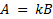# Develop a general mathematical model and implement it on a spreadsheet to find their expected profit.

A (greatly) simplified model of the national economy can be described as follows. The national income is the sum of three components: consumption, investment, and government spending. Consumption is related to the total income of all individuals and to the taxes they pay on income. Taxes depend on total income and the tax rate. Investment is also related to the size of the total income.

a. Use this information to draw an influence diagram by recognizing that the phrase “A is related to B” implies that A influences B in the model.

b. If we assume that the phrase “A is related to B” can be translated into mathematical terms as, where k is some constant, develop a mathematical model for the information provided.

A garage band wants to hold a concert. The expected crowd is 3,000. The average expenditure on concessions is15. Tickets sell for \$10 each, and the band’s profit is 80% of the gate and concession sales, minus a fixed cost of12,000. Develop a general mathematical model and implement it on a spreadsheet to find their expected profit.